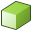# Creating the Waveguide Sections

Create the waveguide sections using two cuboids.

1. Create the first cuboid.
1. On the Construct tab, in the Create solid group, click theCuboid icon.
2. Create the cuboid using the Base centre, width, depth, height definition method.
3. Use the following dimensions:
Dimension Value
Base centre (C) (0, 0, 0)
Width (W) wg_w
Depth (D) 2*wg_w
Height (H) wg_h
Label Cuboid1
2. Create the second cuboid.
1. Create the cuboid using the Base corner, width, depth, height definition method.
2. Use the following dimensions:
Dimension Value
Base corner (C) (wg_w/2, -wg_w/2, 0)
Width (W) wg_w
Depth (D) wg_w
Height (H) wg_h
Label Cuboid2